Report No.
-

Initialising ...

Refine

Initialising ...

Initialising ...

Initialising ...

Initialising ...

Initialising ...

Initialising ...

## Held year of conference

Initialising ...

Save select records

Journal Articles

## Weierstrass function methodology for uncertainty analysis of random media criticality with spectrum range control

Ueki, Taro

Progress in Nuclear Energy, 144, p.104099_1 - 104099_7, 2022/02

Randomized Weierstrass function (RWF) has been under development for evaluating the uncertainty of random media criticality due to the material mixture under disorder. In this work, the modelling capability of RWF is refined so that the spectrum range can be controlled by specifying its lower and upper ends of the frequency domain variable. As a result, it becomes possible to make fair criticality comparison among replicas of random media under inverse power law power spectra. Technically, the infinite sum of trigonometric terms in RWF is extended to cover the arbitrarily low frequency domain and then truncated to finite terms for the sole purpose of spectrum range control. This means that the refinement is free of the convergence issue towards a fractal characteristic of Weierstrass function and thus termed Incomplete Randomized Weierstrass function (IRWF). As a demonstration, a three-dimensional version of IRWF is applied to the mixture of three fuels with different burnups in a water-moderated environment. Monte Carlo criticality calculations are carried out to evaluate the uncertainty of neutron effective multiplication factor due to the indeterminacy of the fuel mixture formation.

Journal Articles

## Effect of moderation condition on neutron multiplication factor distribution in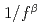random media

Araki, Shohei; Yamane, Yuichi; Ueki, Taro; Tonoike, Kotaro

Nuclear Science and Engineering, 195(10), p.1107 - 1117, 2021/10

Times Cited Count：0 Percentile：0.01(Nuclear Science & Technology)

Criticality control of random media such as fuel debris is one of the most important safety issues in post-accident management.spectrum randomizing model is expected to simulate such random media because it is well known that thenoise can describe a diverse range of random and disordered natural phenomena. In this paper, we focused on the relationship between the multiplication factor and moderation condition in therandom media. A number of random media were realized with thespectrum randomizing model that is based on the Randomized Weierstrass function (RWF). The volume ratio of concrete to fuel was adopted as an index for the moderation condition. The multiplication factors were calculated with a two-energy group Monte Carlo calculation. The calculation results were analyzed by using variance, skewness, and kurtosis. Those statistical parameters had an extreme value around the optimum moderation condition. This result suggested that it is possible to predict the rough trend of variation range, distortion, and outlier of multiplication factors in therandom media.

Journal Articles

## Monte Carlo criticality calculation of random media formed by multimaterials mixture under extreme disorder

Ueki, Taro

Nuclear Science and Engineering, 195(2), p.214 - 226, 2021/02

Times Cited Count：1 Percentile：39.17(Nuclear Science & Technology)

A dynamical system under extreme physical disorder has the tendency of evolving toward the equilibrium state characterized by an inverse power law power spectrum. In this paper, a practically implementable three-dimensional model is proposed for the random media formed by multi-materials mixture under such a power spectrum using a randomized form of Weierstrass function, its extension covering the white noise, and partial volumes pairings of constituent materials. The proposed model is implemented in the SOLOMON Monte Carlo solver with delta tracking. Two sets of numerical results are shown using the JENDL-4 nuclear data libraries.

Journal Articles

## Judgment on convergence-in-distribution of Monte Carlo tallies under autocorrelation

Ueki, Taro

Nuclear Science and Engineering, 194(6), p.422 - 432, 2020/06

Times Cited Count：0 Percentile：0.01(Nuclear Science & Technology)

In Monte Carlo criticality calculation, the convergence-in-distribution check of the sample mean of tallies can be approached in terms of the influence range of autocorrelation. In this context, it is necessary to evaluate the attenuation of autocorrelation coefficients over lags. However, in just one replica of calculation, it is difficult to accurately estimate small ACCs at large lags because of the comparability with statistical uncertainty. This paper proposes a method to overcome such an issue. Its essential component is the transformation of a standardized time series of tallies so that the resulting series asymptotically converges in distribution to Brownian motion. The convergence-in-distribution check is constructed based on the independent increment property of Brownian motion. The judgment criterion is set by way of the spectral analysis of fractional Brownian motion. Numerical results are demonstrated for extreme and standard types of criticality calculation.

Journal Articles

## Effect of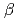on effective multiplication factor in 1/f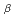spectrum random system

Araki, Shohei; Yamane, Yuichi; Ueki, Taro; Tonoike, Kotaro

Proceedings of International Conference on the Physics of Reactors; Transition To A Scalable Nuclear Future (PHYSOR 2020) (USB Flash Drive), 8 Pages, 2020/03

We investigated thedependence of the effective multiplication factor (k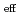) in the 1/fnoise model. We conducted the two-group Monte Carlo calculations. We found that the standard deviation of the kdistribution showed the positive correlation with thevalue because the spatial distribution of the fuel became less uniform as thevalue increased.

Journal Articles

## Solomon; A Monte Carlo solver for criticality safety analysis

Nagaya, Yasunobu; Ueki, Taro; Tonoike, Kotaro

Proceedings of 11th International Conference on Nuclear Criticality Safety (ICNC 2019) (Internet), 9 Pages, 2019/09

A new Monte Carlo solver Solomon has been developed for the application to fuel-debris systems. It is designed not only for usual criticality safety analysis but also for criticality calculations of damaged reactor core including fuel debris. This paper describes the current status of Solomon and demonstrates the applications of the randomized Weierstrass function (RWF) model and the RWF model superimposed voxel geometry.

Journal Articles

## Universal methodology for statistical error and convergence of correlated Monte Carlo tallies

Ueki, Taro

Nuclear Science and Engineering, 193(7), p.776 - 789, 2019/07

Times Cited Count：4 Percentile：68.11(Nuclear Science & Technology)

It is known that the convergence of standardized time series (STS) to Brownian bridge yields standard deviation estimators of the sample mean of correlated Monte Carlo tallies. In this work, a difference scheme based on a stochastic differential equation is applied to STS in order to obtain a new functional statistic (NFS) that converges to Brownian motion (BM). As a result, statistical error estimation improves twofold. First, the application of orthonormal weighting to NFS yields a new set of asymptotically unbiased standard deviation estimators of sample mean. It is not necessary to store tallies once the updating of estimator computation is finished at each generation. Second, it becomes possible to assess the convergence of sample mean in an assumption-free manner by way of the comparison of power spectra of NFS and BM. The methodology is demonstrated for three different types of problems encountered in Monte Carlo criticality calculation.

Journal Articles

## Continuous energy Monte Carlo criticality calculation of random media under power law spectrum

Ueki, Taro

Proceedings of International Conference on Mathematics and Computational Methods applied to Nuclear Science and Engineering (M&C 2019) (CD-ROM), p.151 - 160, 2019/00

A dynamical system under extreme physical disorder has the tendency of evolving toward the equilibrium state characterized by an inverse power law spectrum. In this paper, the author proposes a practically implementable modeling of random media under such a spectrum using a randomized form of the Weierstrass function. The proposed modeling is demonstrated by the continuous energy Monte Carlo particle transport with delta tracking for the criticality calculation of a randomized version of the Topsy spherical core in International Criticality Safety Benchmark Evaluation Project.

Journal Articles

## Monte Carlo criticality analysis of random media under bounded fluctuation driven by normal noise

Ueki, Taro

Journal of Nuclear Science and Technology, 55(10), p.1180 - 1192, 2018/10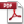AA2018-0157.pdf:1.15MB

Times Cited Count：3 Percentile：45.99(Nuclear Science & Technology)

In Monte Carlo criticality analysis under material distribution uncertainty, it is necessary to evaluate the response of neutron effective multiplication factor (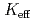) to the space-dependent random fluctuation of volume fractions within a prescribed bounded range. Normal random variables, however, cannot be used in a straightforward manner since the normal distribution has infinite tails. To overcome this issue, a methodology has been developed via forward-backward-superposed reflection Brownian motion (FBSRBM). Here, the forward-backward superposition makes the variance of fluctuation spatially constant and the reflection Brownian motion confines the fluctuation driven by normal noise in a bounded range. FBSRBM was implemented using Karhunen-Loeve expansion and applied to the fluctuation of volume fractions in a model of UO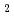-concrete media with stainless steel.

Journal Articles

## A Power spectrum approach to tally convergence in Monte Carlo criticality calculation

Ueki, Taro

Journal of Nuclear Science and Technology, 54(12), p.1310 - 1320, 2017/12AA2017-0413.pdf:1.05MB

Times Cited Count：6 Percentile：65.17(Nuclear Science & Technology)

In Monte Carlo criticality calculation, confidence interval estimation is based on the central limit theorem (CLT) for a series of tallies. A fundamental assertion resulting from CLT is the convergence in distribution (CID) of the interpolated standardized time series (ISTS) of tallies. In this work, the spectral analysis of ISTS has been conducted in order to assess the convergence of tallies in terms of CID. Numerical results indicate that the power spectrum of ISTS is equal to the theoretically predicted power spectrum of Brownian motion for effective neutron multiplication factor; on the other hand, the power spectrum of ISTS for local power fluctuates wildly while maintaining the spectral form of fractional Brownian motion. The latter result is the evidence of a case where a series of tallies is away from CID, while the spectral form supports normality assumption on the sample mean.

Journal Articles

## Spectral analysis for convergence assessment in Monte Carlo criticality calculation

Ueki, Taro

Proceedings of International Conference on Mathematics & Computational Methods Applied to Nuclear Science & Engineering (M&C 2017) (USB Flash Drive), 6 Pages, 2017/04

In Monte Carlo criticality calculation, the formation of a confidence interval is based on the central limit theorem for a series of tallies from generations in equilibrium. A fundamental assertion of the theorem is the convergence in distribution (CID) of an interpolated standardized time series (ISTS) of tallies. This article reports a spectral analysis approach to ISTS in order to assess the convergence of tallies in terms of CID. Numerical results are demonstrated for a preliminary model of uranium-concrete debris.

Journal Articles

## Monte Carlo criticality analysis under material distribution uncertainty

Ueki, Taro

Journal of Nuclear Science and Technology, 54(3), p.267 - 279, 2017/03

Times Cited Count：5 Percentile：58.67(Nuclear Science & Technology)

Analysis framework under material distribution uncertainty is investigated for the Monte Carlo (MC) criticality calculation of continuously mixed media formed via molten core concrete interaction. Deterministic trigonometric functions and randomized Weierstrass functions are utilized to represent the spatially continuous variation. Numerical results indicate that the effective multiplication factor (k) under random spatial variation can depart significantly from the kof a reference uniform medium. It is also shown that the deterministic modeling provides an upper-bound measure for extreme results from random realizations.

Journal Articles

## Fractal dimension analysis for run length diagnosis of Monte Carlo criticality calculation

Ueki, Taro

Journal of Nuclear Science and Technology, 53(3), p.312 - 322, 2016/03

Times Cited Count：5 Percentile：54.39(Nuclear Science & Technology)

In Monte Carlo criticality calculation (MCCC), each output quantity of interest is a series of tallies under autocorrelation. As a consequence from the functional central limit theorem, the stepwise interpolation of standardized tallies (SIST) converges in distribution to Brownian bridge (BB). Here, the standardization of tallies is a functional version of the statistic in the central limit theorem with the sample mean at each generation and the true mean replaced by the sample mean at the final generation. Fractional Brownian motion (FBM) is a family of stochastic processes and assumes a unique value of the box counting dimension (BCD) in fractal geometry. This work shows that the BCD of SIST is an effective diagnostic measure for the run length of MCCC. The sufficiency of the number of generations run can be judged by relating the BCD of FBM to that of BB. Numerical results are presented for power distribution tallies of a pressurized water reactor and the effective multiplication factor () tallies of the criticality problem by D. Mennerdahl.

Journal Articles

## Brownian bridge and statistical error estimation in Monte Carlo reactor calculation

Ueki, Taro

Journal of Nuclear Science and Technology, 50(8), p.762 - 773, 2013/08

Times Cited Count：4 Percentile：37.15(Nuclear Science & Technology)

Brownian bridge (BB) is an effective vehicle in processing an output series in Monte Carlo (MC) simulation. However, most estimators based on BB cost the capability of on-the-fly monitoring. Here, on-the-fly implies that statistical error can be computed at every generation except some initial generations. In this work, on-the-fly estimation of standard deviation by way of BB, which maintains a fixed storage size of tallies, has been investigated within a framework of the iterated integration of simulation output (IISO). Numerical tests on the MC power distribution calculation of a pressurized water reactor core reveal that the IISO approach with a relatively few number of integrations performs fairly well on average. The bias of statistical error can be managed to be about 10% or less.

Journal Articles

## Monte Carlo criticality calculation under extreme condition

Ueki, Taro

Journal of Nuclear Science and Technology, 49(12), p.1134 - 1143, 2012/12

Times Cited Count：11 Percentile：69.17(Nuclear Science & Technology)

Autocorrelation and bias characteristics in the Monte Carlo criticality calculation have been examined for a certain type of extreme problems. Possibility is shown that the bias of the effective multiplication factor can become larger than -0.1 in magnitude for population size as large as 10,000 starter particles per generation. This bias dramatically decreases if the number of starter particles per generation is large enough so that the noise propagation of a source distribution becomes linear. Furthermore, under this linearity, the bias correction is demonstrated to be an estimation problem of autocorrelation. Therefore, the linearity diagnosis of the noise propagation should be made available in the Monte Carlo criticality codes in public release in order to encompass analysis scenarios beyond normal circumstances.

Journal Articles

## New result in the production and decay of an isotope,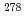113 of the 113th element

Morita, Kosuke*; Morimoto, Koji*; Kaji, Daiya*; Haba, Hiromitsu*; Ozeki, Kazutaka*; Kudo, Yuki*; Sumita, Takayuki*; Wakabayashi, Yasuo*; Yoneda, Akira*; Tanaka, Kengo*; et al.

Journal of the Physical Society of Japan, 81(10), p.103201_1 - 103201_4, 2012/10

Times Cited Count：142 Percentile：97.35(Physics, Multidisciplinary)

An isotope of the 113th element,113, was produced in a nuclear reaction with a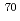Zn beam on a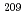Bi target. We observed six consecutive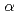decays following the implantation of a heavy particle in nearly the same position in the semiconductor detector, in extremely low background condition. The fifth and sixth decays are fully consistent with the sequential decays of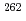Db and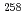Lr both in decay energies and decay times. This indicates that the present decay chain consisted of113,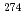Rg (Z = 111),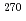Mt (Z = 109),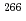Bh (Z = 107),Db (Z = 105), andLr (Z = 103) with firm connections. This result, together with previously reported results from 2004 and 2007, conclusively leads the unambiguous production and identification of the isotope113, of the 113th element.

Journal Articles

## An Orthonormally weighted standardized time series for the error estimation of local tallies in Monte Carlo criticality calculation

Ueki, Taro

Nuclear Science and Engineering, 171(3), p.220 - 230, 2012/07

Times Cited Count：11 Percentile：69.17(Nuclear Science & Technology)

An orthonormally weighted standardized time series (OWSTS) was investigated for the statistical error estimation in the Monte Carlo calculation of local tallies. Unlike the original implementation of a standardized time series, the computation of standard deviation via OWSTS can be made free of the grouping of iteration cycles into batches. Its characteristic aspect is the application of an arbitrary number of weighting functions to a standardized series of tallies such that asymptotically independent and unbiased estimates are produced based on the statistics of Brownian bridge. In the present work, a trigonometric set of weighting functions is extended and applied to local power tallies in a pressurized water reactor. Numerical results are demonstrated that the OWSTS error estimation is unbiased for a sufficiently large number of iteration cycles.

Journal Articles

##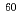Co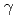-ray irradiation effects on pentacene-based organic thin film transistors

Cai, L.*; Hirao, Toshio; Yano, Hiroaki*; Duan, Z.*; Takayanagi, Yutaro*; Ueki, Hideharu*; Oshima, Takeshi; Nishioka, Yasushiro*

Materials Science Forum, 687, p.576 - 579, 2011/06

Times Cited Count：3 Percentile：85.69

no abstracts in English

Journal Articles

## Histrory of benchmark experiment analysis with Monte Carlo mehtod for nuclear data reliability evaluation

Sakurai, Kiyoshi; Ueki, Kotaro*

Nihon Genshiryoku Gakkai-Shi, 43(4), p.351 - 352, 2001/04

no abstracts in English

Journal Articles

## Education program for criticality safety with Monte Carlo method

Sakurai, Kiyoshi; Ueki, Kotaro*; Naito, Yoshitaka*

Nihon Genshiryoku Gakkai-Shi, 43(3), p.219 - 220, 2001/03

no abstracts in English